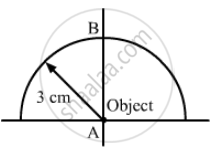Department of Pre-University Education, KarnatakaPUC Karnataka Science Class 12
Advertisement Remove all ads

# A Paperweight in the Form of a Hemisphere of Radius 3.0 Cm is Used to Hold Down a Printed Page. an Observer Looks at the Page Vertically Through the - Physics

Sum

A paperweight in the form of a hemisphere of radius 3.0 cm is used to hold down a printed page. An observer looks at the page vertically through the paperweight. At what height above the page will the printed letters near the centre appear to the observer?

Advertisement Remove all ads

#### Solution

Given:
Radius of the paperweight (R) = 3 cm
Refractive index of the paperweight (μ2) = 3/2
Refractive index of the air (μ1) = 1In the first case, the refraction is at A.
u = 0 and R = ∞
We know:

$\frac{\mu_2}{v} - \frac{\mu_1}{u} = \frac{\mu_2 - \mu_1}{R}$

$\Rightarrow \frac{\frac{3}{2}}{v} - \frac{1}{0} = \frac{\mu_2 - \mu_1}{\infty}$

$\Rightarrow v = 0$
Therefore, the image of the letter is formed at the point.
For the second case, refraction is at point B.
Here,
Object distance, = −3 cm
= − 3 cm
μ1 = 3/2
μ2 = 1
Thus, we have:

$\frac{\mu_2}{v} - \frac{\mu_1}{u} = \frac{\mu_2 - \mu_1}{R}$

$\frac{1}{v} - \frac{3}{2 \times ( - 3)} = \frac{1 - \frac{3}{2}}{- 3}$

$\Rightarrow \frac{1}{v} + \frac{3}{2 \times 3} = \frac{1}{6}$

$\Rightarrow \frac{1}{v} = \frac{1}{6} - \frac{1}{2}$

$\Rightarrow v = - 3 \text{ cm }$
Hence, there will be no shift in the final image.

Is there an error in this question or solution?
Advertisement Remove all ads

#### APPEARS IN

HC Verma Class 11, 12 Concepts of Physics 1
Chapter 18 Geometrical Optics
Q 44 | Page 415
Advertisement Remove all ads
Advertisement Remove all ads
Share
Notifications

View all notifications

Forgot password?